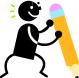Using Fact Family Math Mats

Domino Fact Families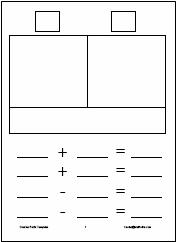Dominoes can be used to introduce students to fact families. Students need a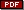Domino Facts Template inserted in a sheet protector, dry erase marker, and some dominoes for this activity. The student selects a domino and draws it on the template (or uses counters to build the domino). He/she then counts the number of dots on each side of the domino, writing the numbers in the squares above the domino sides. The student figures out the total number of dots and writes this number in the rectangle below the domino. These three numbers are the number family the students will use to write the 4 number sentences for that fact family.

Alternately, the teacher may display aDomino Flash Card and have the whole class use the same domino for the introductory activity. In this case, the teacher should use an overhead of theDomino Facts Template. NOTE: inserting the overhead in a sheet protector allows the teacher to use dry erase markers and preserves the life of the overhead. For storage, many teachers elect to keep these often-used overheads in a binder.

Differentiation: Vary the complexity of the dominoes students use to accommodate the varied needs of learners in the class.

Center Activity: Make the materials available in the math center so that students practice fact families on a regular basis.

Do Now! Transition Activity: Many teachers opt to have students keep theDomino Facts Template in their desks so that they can use this activity as a daily part of math class, beginning or ending math class with fact family practice.

Fact FamiliesAfter students have lots of experience writing number sentences for mat activities, students are ready to work on fact families, a series of four related facts for a given set of three numbers. Students need aFact Family Template inserted in a sheet protector and a dry erase marker for this practice.

This template is designed to reinforce the notion that families live together in a house. The smaller two addends are written in each window. The larger number (the sum) is written on the door. Students use the 3 family numbers to write 2 addition sentences and 2 subtraction sentences.

Students will need lots of modeling and guided practice to transition to writing the numerals in the correct spots and using the numbers correctly to form the four number sentences in the fact family. Students usually have difficulty remembering to use the larger number first in the subtraction sentences. Some students benefit from reminders to use the door number first.

Checking their work: All students should be reminded to check their work to make sure that each number sentence is correct and that they have only used the three numbers in the number fact family. Teachers may ask students to check off or color in a bush for each fact that they check, using the bushes as a prompt to self-monitor and check their work.

Center Activity: Provide templates and dry erase markers at the center. Write fact families on index cards for student use. Be sure to include some mixed-up families where the sum is in the middle, for instance, so that students have practice sorting out the numbers to use them correctly in the template and in the number sentences.

Triangle Fact Families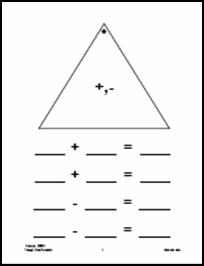Many teachers use the triangle facts to practice addition and subtraction facts. The beauty of these cards, as opposed to traditional flash cards, is that each card has the three numbers of a fact family. By varying what number the teacher covers, the same card can be used for addition or subtraction fact practice. Note that the larger number is at the top of the triangle and has a dot next to it to indicate the sum number.

Students need aTriangle Cards Template inserted in a sheet protector and a dry erase marker for this fact family practice.

Guided Practice: Hold up a triangle card or use an overhead of theTriangle Cards Template to write in the three numbers. Students copy the numbers onto their templates and then write the four number sentences for that fact family. The teacher circulates around the room, monitoring student progress and assisting students, as needed. Students may be asked to pair-check their work, as a variation.

Individual practice: Give each student a triangle card(s) and ask them to use that card to write four number sentences for that fact family. Students may exchange cards after their work is checked for accuracy.

Challenge: Write in only two of the three numbers and challenge students to figure out the third number and then write the four number sentences for that fact family. Be sure to have students explain how they figured out the missing number as this increases the problem-solving repertoire of all students in the class.

Mathwire.com: Math Mats Resources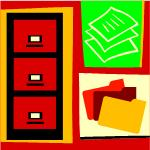This series of pages is designed as a resource to teachers as they differentiate instruction for varied learners in the class. Each page discusses how to use the set of mats to develop mathematical understanding and support student growth.